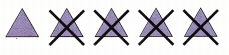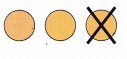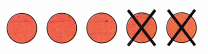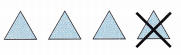# McGraw Hill Math Grade 1 Chapter 3 Lesson 1 Answer Key Subtraction Facts Through 6

All the solutions provided in McGraw Hill Math Grade 1 Answer Key PDF Chapter 3 Lesson 1 Subtraction Facts Through 6 as per the latest syllabus guidelines.

## McGraw-Hill Math Grade 1 Answer Key Chapter 3 Lesson 1 Subtraction Facts Through 6

Subtract

Question 1.By subtracting 2 from 4 we get 2.

Question 2.5 – 4 = ___
By subtracting 4 from 5 we get 1.

Question 3.3 – 1 = ___
3 – 1 = 2
By subtracting 1 from 3 we get 2.

Question 4.6 – 3 = _____
6 – 3 = 3
By subtracting 3 from 6 we get 3.

Question 5.5 – 2 = ___